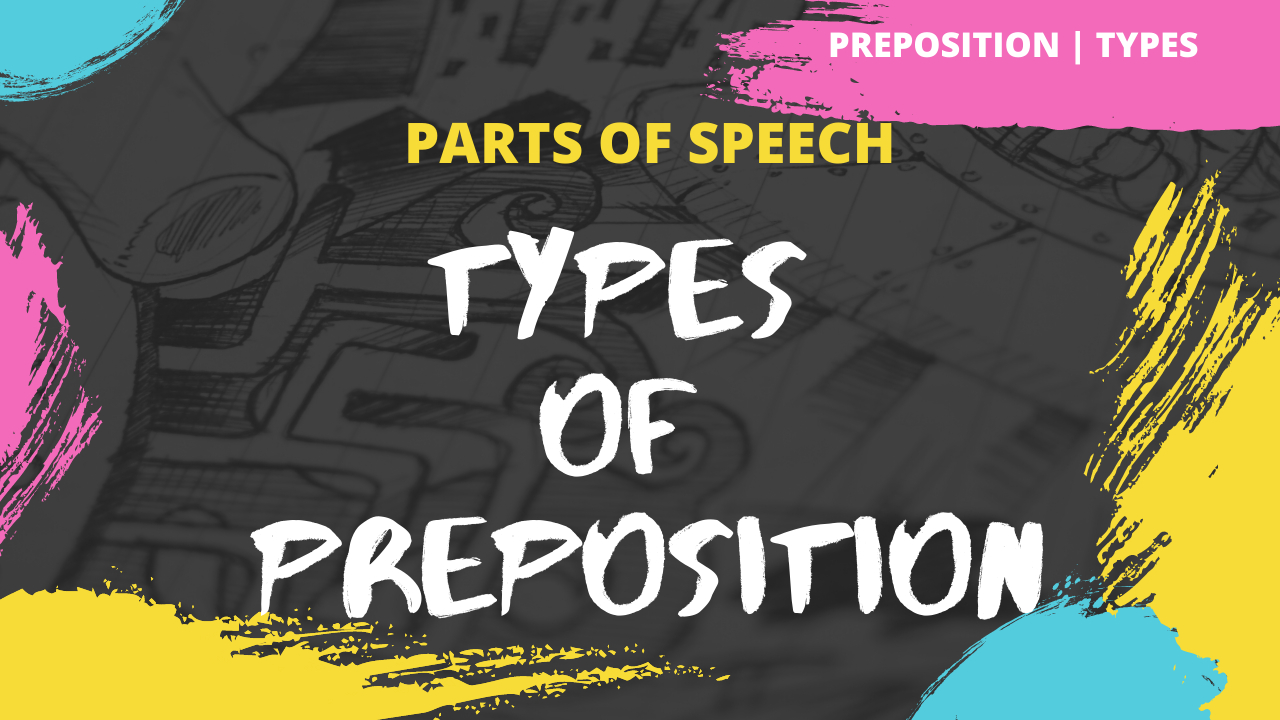## What is Preposition | Types of Prepositions

#### What is a Preposition?

The link means they provide a relationship between a noun and other words in the sentence and thus make the sentence complete.
These other words may be a noun, verb, or adjective.

Let’s understand it with an example.
This cat is running and now where is it sitting?
The cat is sitting under the table. Here, under is used to link table which is a noun and sitting placement of cat.
And hence under is a preposition here.

#### Types of Prepositions

These are divided into 3 types depending on three situations.

1. Preposition of Time
2. Preposition of Place
3. Preposition of Direction
1. Time – The preposition that describes when an event happened is defined as the preposition of time.
For example: in, on, at, before, after.
Let’s look into some sentence examples to make things clear.
• My birthday falls in January.
• My vacation ends on Monday.
• It gets cold at night.

Remember that this preposition links ‘when one thing happened’ in context to others. Now, let’s look into the next one.

2. Place – The preposition that describes where anything is in context to another, is defined as the preposition of place.
For example: under, above, in, on, at.

Now, you might be thinking that we had ‘in, on, at’ as a preposition of time and here also the same.
But please note they are used in a different meaning. Let’s see some sentence examples to make things clear.

• The books are on the table. Now, where are the books placed? The books are placed on the table.
Here, ‘ON’ describes the link between the table and books. Hence it is the preposition of place.
• The plane is just below the cloud. Here ‘below’ is the linking word.
Remember, this preposition provides where the thing is concerning others. Now, let’s look into the next type.

3. Direction – The preposition that describes where to go or where to put something is called the preposition of direction.
For example: to, into, off, from.

Let me give you some sentence examples to make it clear.

• We went to London last week.
• He went up the hill.
Remember, this preposition provides in which direction the thing is concerning others.

Now, let’s look into one more term related to preposition.

#### Prepositional Phrase

What is a phrase?

It is a group of words. And what is Preposition phrase
It is a group of words containing preposition.

Let me give you some examples of it.

• The rabbit hopped through the garden. Here, through is the preposition, and ‘through the garden’ is the whole prepositional phrase.
• The balloon drifted up the stairs. Here, up is the preposition, and ‘up the stairs’ is the whole prepositional phrase.
• The tiger crept slowly over the grass. Here, over is the main proposition, and ‘over the grass’ is the prepositional phrase.

So, remember that prepositional phrases are a group of words containing preposition.

Writing Articles :

See more such videos on YouTube Channel